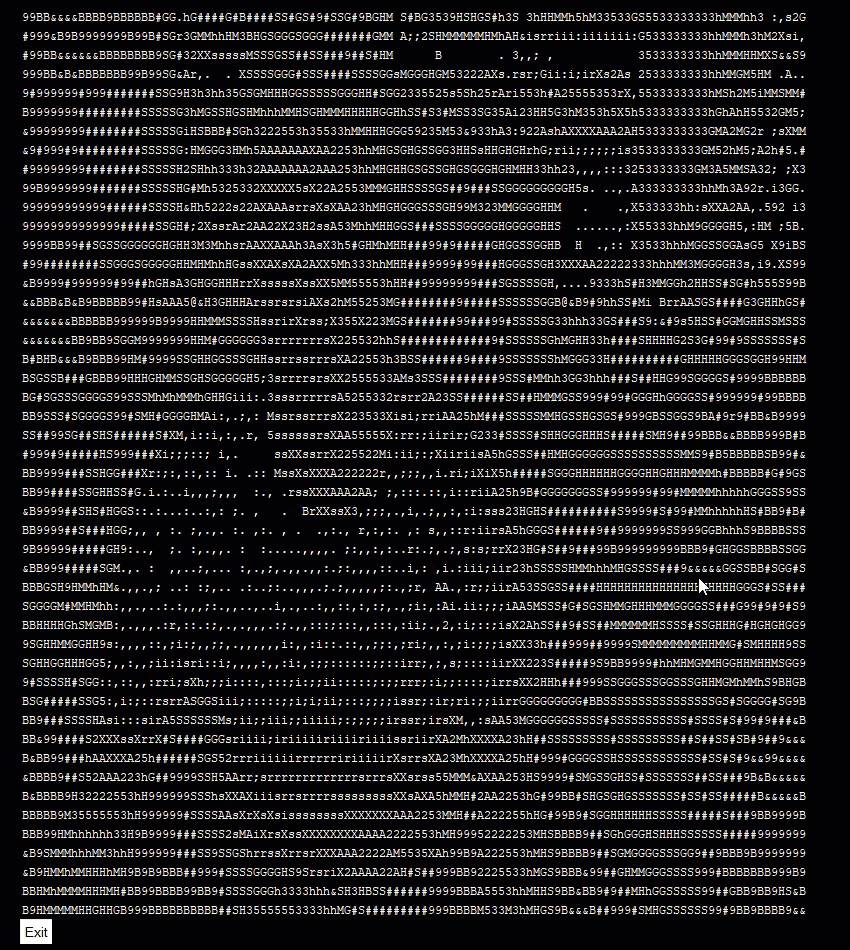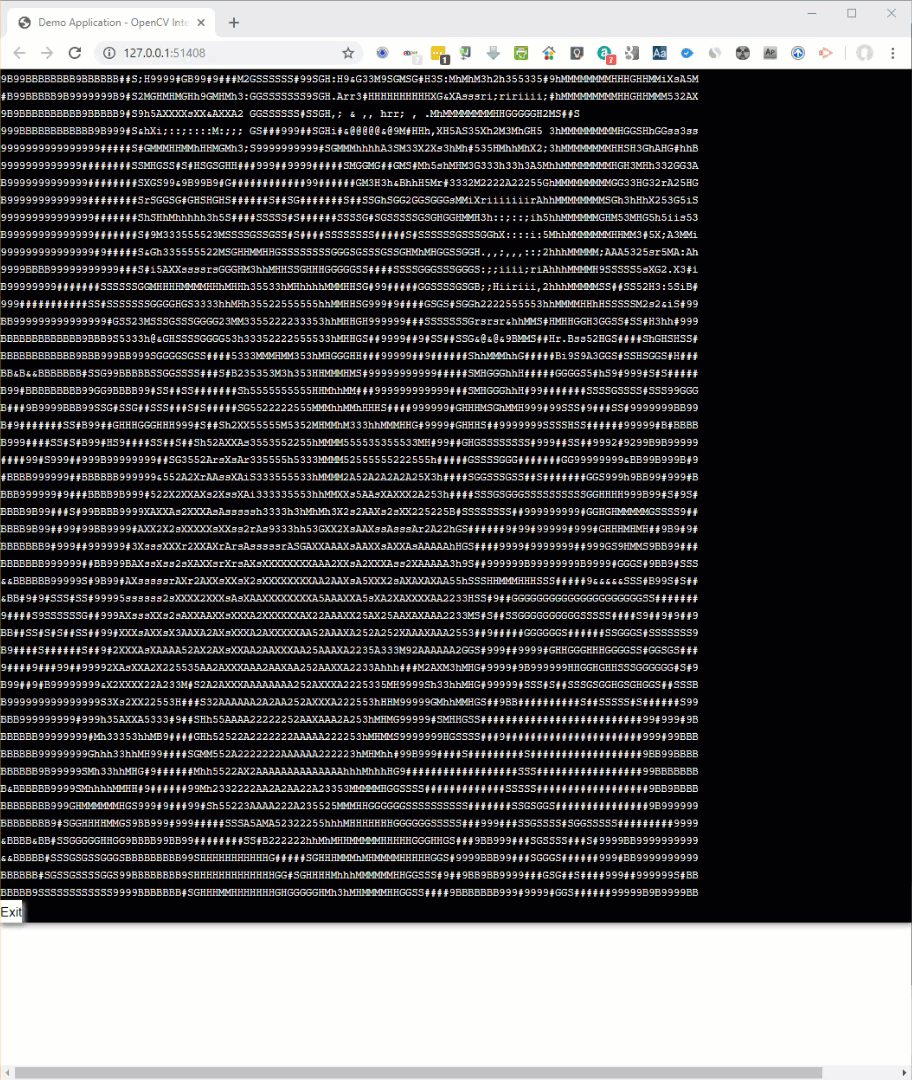{{ message }}

Instantly share code, notes, and snippets.

# cdiener/asciinator.py

Last active May 26, 2021
Convert image to ascii art
This file contains bidirectional Unicode text that may be interpreted or compiled differently than what appears below. To review, open the file in an editor that reveals hidden Unicode characters. Learn more about bidirectional Unicode characters
 import sys; from PIL import Image; import numpy as np chars = np.asarray(list(' .,:;irsXA253hMHGS#9B&@')) if len(sys.argv) != 4: print( 'Usage: ./asciinator.py image scale factor' ); sys.exit() f, SC, GCF, WCF = sys.argv, float(sys.argv), float(sys.argv), 7/4 img = Image.open(f) S = ( round(img.size*SC*WCF), round(img.size*SC) ) img = np.sum( np.asarray( img.resize(S) ), axis=2) img -= img.min() img = (1.0 - img/img.max())**GCF*(chars.size-1) print( "\n".join( ("".join(r) for r in chars[img.astype(int)]) ) )

###codedude commented Jun 16, 2016

 Thanks, it works great ;)

###stratosgear commented Nov 16, 2016

 Annotated version: https://gist.github.com/cdiener/10567484

###peter-rusin commented Nov 18, 2017

 Thanks!

###Schizo commented Nov 29, 2017

 you can do all one line when you fill it up with `;`

###liyunze-coding commented Jun 4, 2019

 Sorry, I'm kinda new to this, but how do you exactly make this work while you're running it?

###MikeTheWatchGuy commented Aug 14, 2019

 OMG Thank you for this!!!!! I was able to use it with my GUI package and run it in a GUI window (tkinter based) and changed a line of code and ran it in a Browser (Remi based). And, it was trivial to add in your code. It was all done in 25 lines of code thanks your magic! Thank you!!! Here's the PySimpleGUI one (tkinter)Here's the PySimpleGUIWeb versionSorry that I hacked up your code a bit, but it wasn't THAT bad. Can I put the code here? I'll move it if asked. Just don't want to fart around with a Gist and it does show one way of integrating your code into something bigger perhaps ```from PIL import Image; import numpy as np import PySimpleGUI as sg import cv2 # The magic bits that make the ASCII stuff work shamelessly taken from https://gist.github.com/cdiener/10491632 chars = np.asarray(list(' .,:;irsXA253hMHGS#9B&@')) SC, GCF, WCF = .1, 1, 7/4 sg.ChangeLookAndFeel('Black') # make it look cool # define the window layout NUM_LINES = 48 # number of lines of text elements. Depends on cameras image size and the variable SC (scaller) layout = [*[[sg.T(i, size=(115,1), font=('Courier', 12), key='_OUT_'+str(i))] for i in range(NUM_LINES)], [ sg.Button('Exit')]] # create the window and show it without the plot window = sg.Window('Demo Application - OpenCV Integration', layout, location=(800,400), no_titlebar=True, grab_anywhere=True, element_padding=(0,0)) # ---===--- Event LOOP Read and display frames, operate the GUI --- # cap = cv2.VideoCapture(0) # Setup the OpenCV capture device (webcam) while True: event, values = window.Read(timeout=0) if event in ('Exit', None): break ret, frame = cap.read() # Read image from capture device (camera) img = Image.fromarray(frame) # create PIL image from frame # More magic that coverts the image to ascii S = (round(img.size * SC * WCF), round(img.size * SC)) img = np.sum(np.asarray(img.resize(S)), axis=2) img -= img.min() img = (1.0 - img / img.max()) ** GCF * (chars.size - 1) # "Draw" the image in the window, one line of text at a time! for i, r in enumerate(chars[img.astype(int)]): window.Element('_OUT_'+str(i)).Update("".join(r)) window.Close()```